# Perimeter Calculator

Calculate the perimeter of a shape by selecting a shape and entering your measurements in any unit, metric or imperial. We’ve included the formulas for each shape below.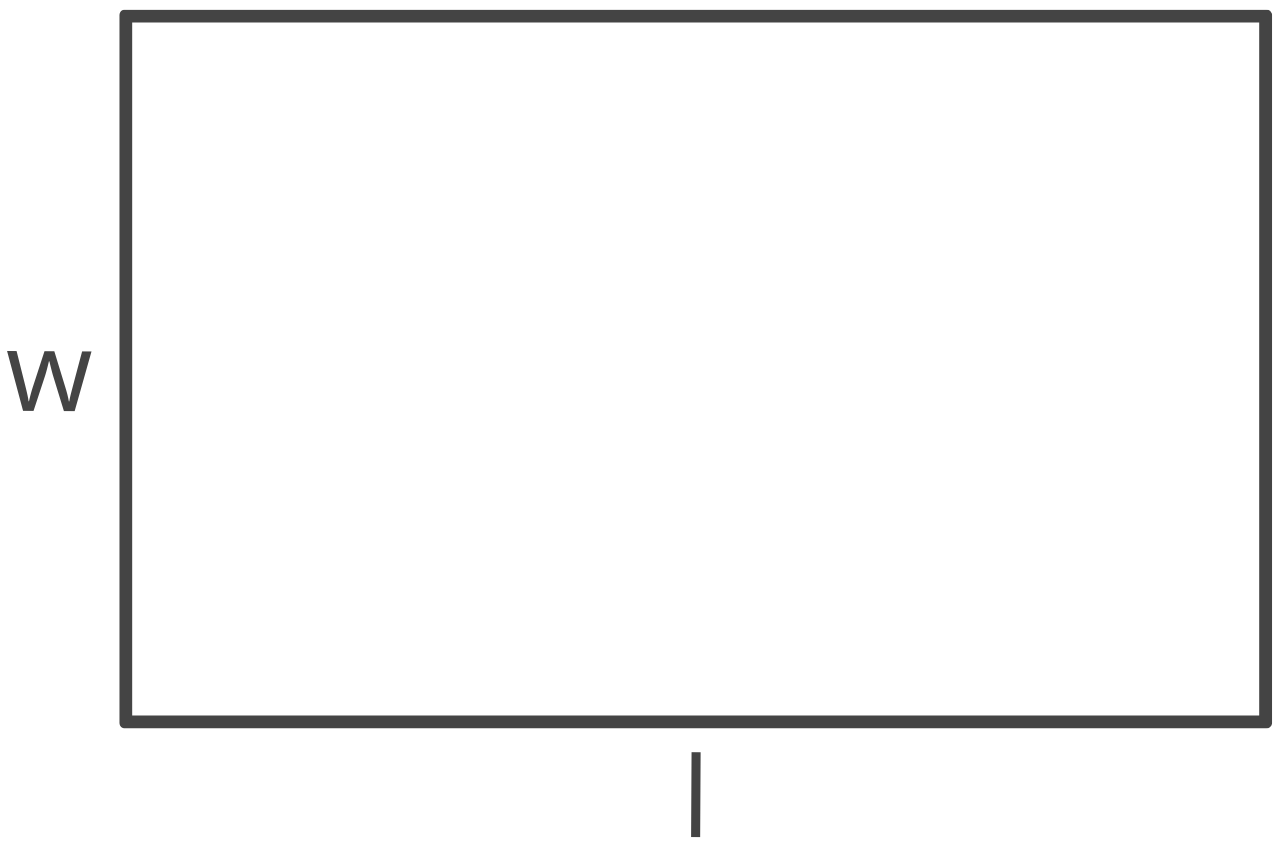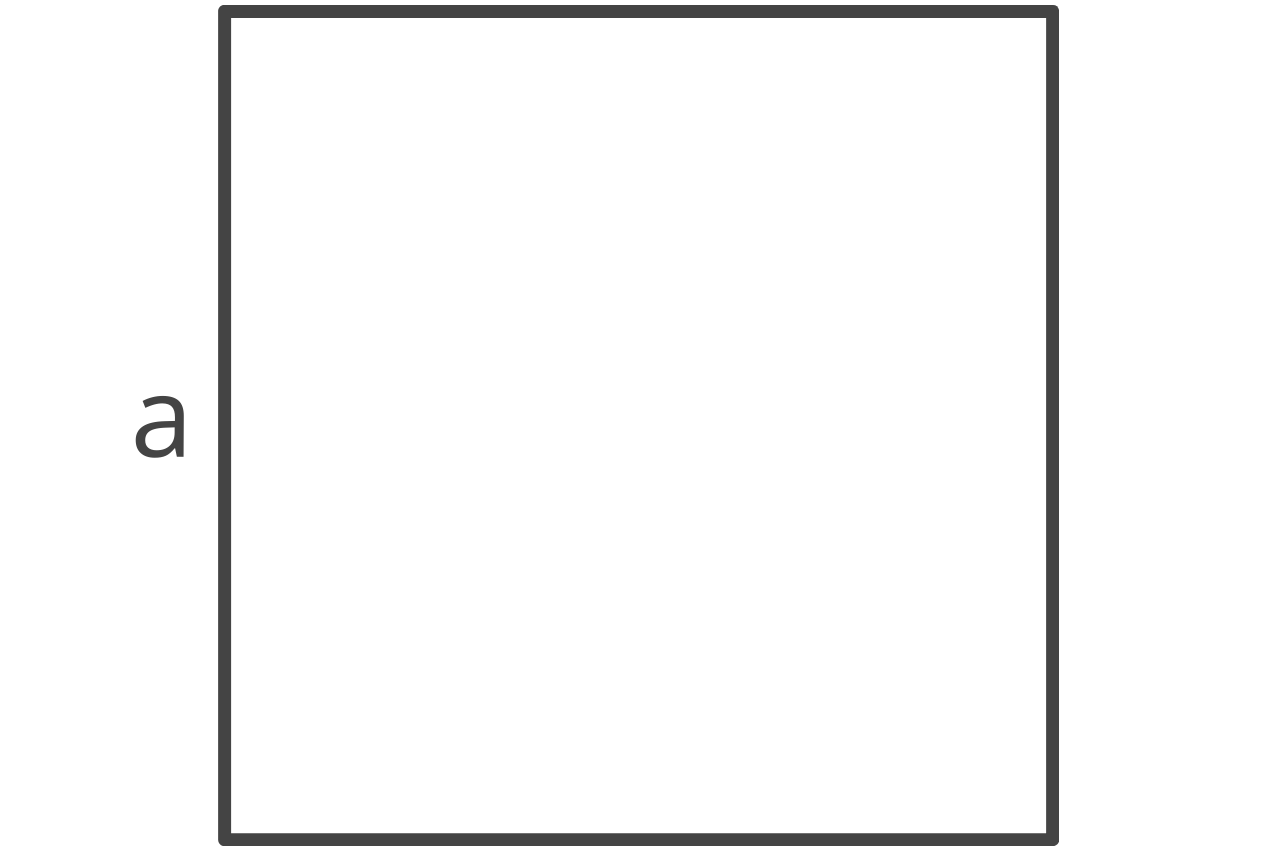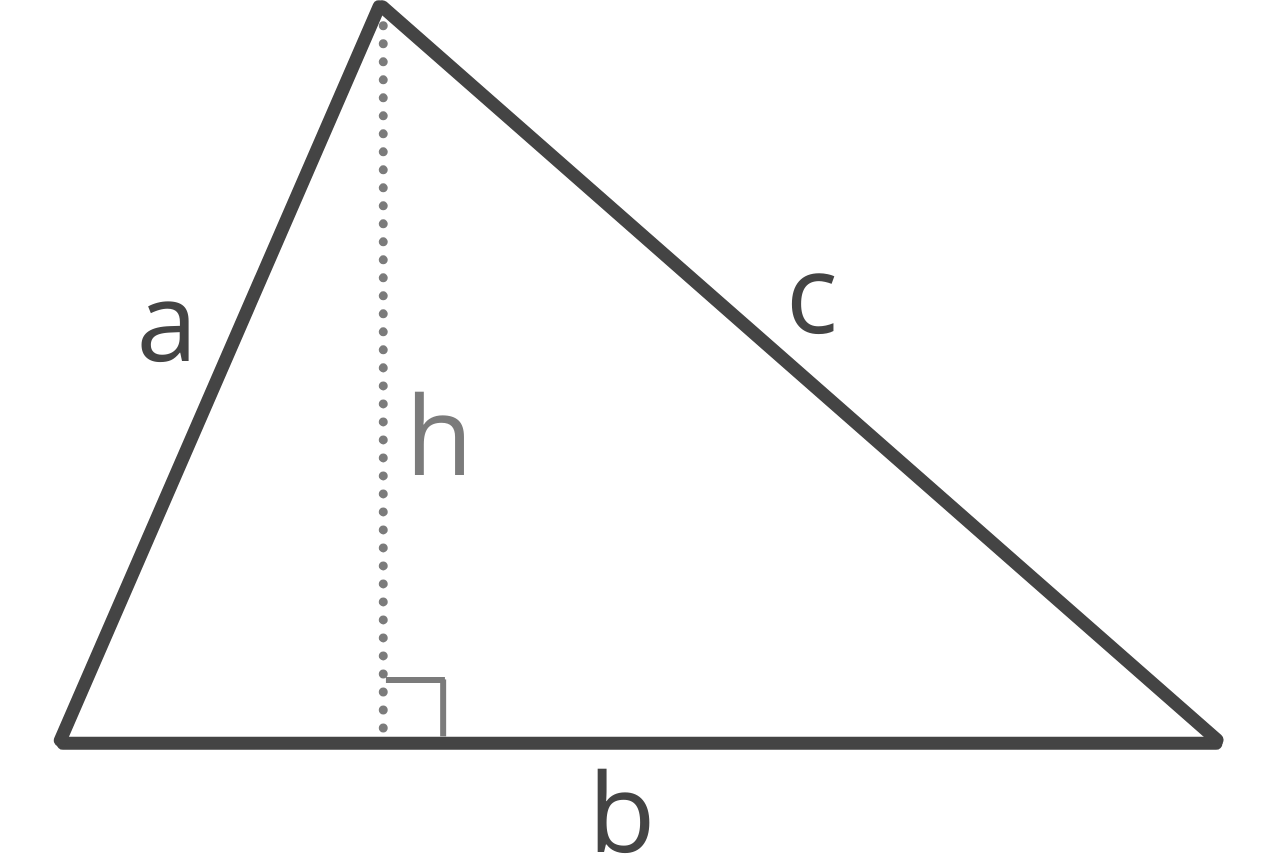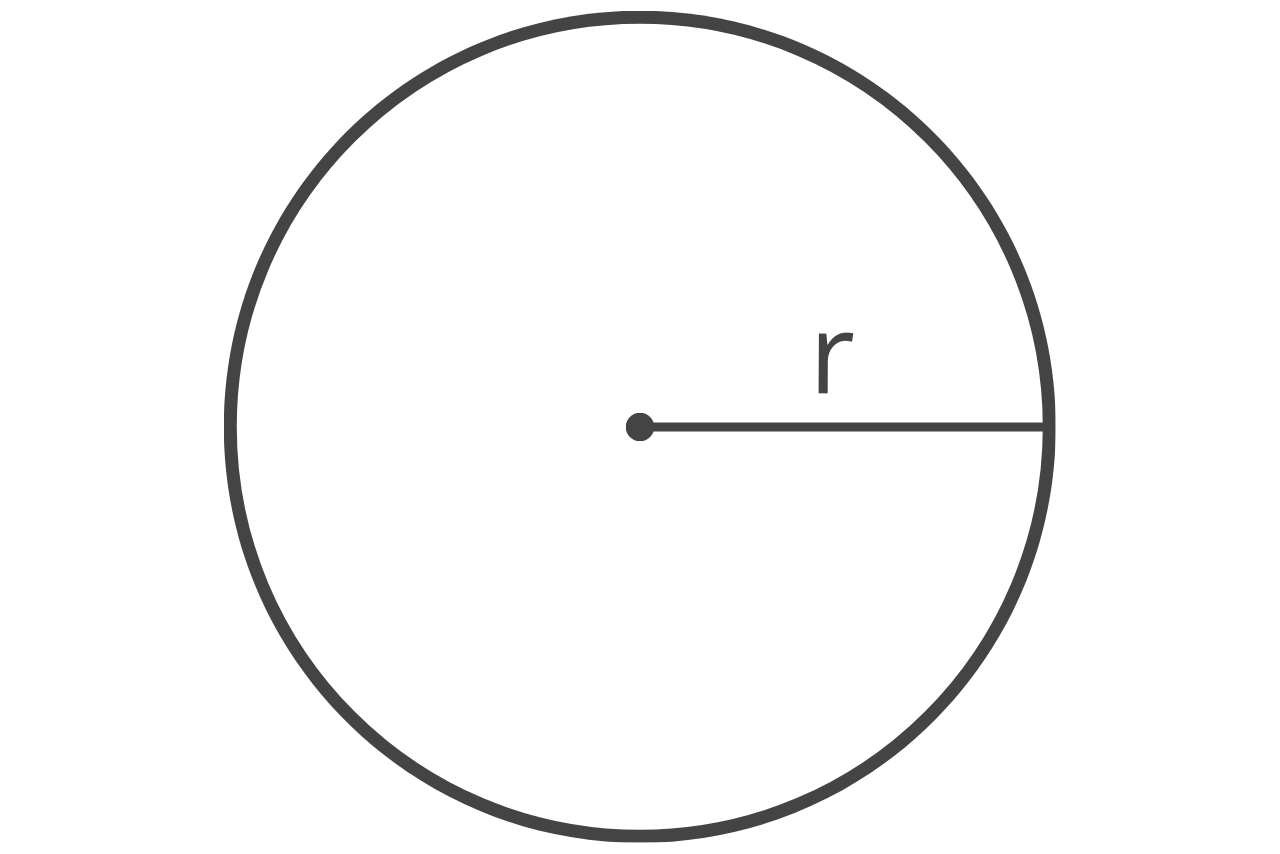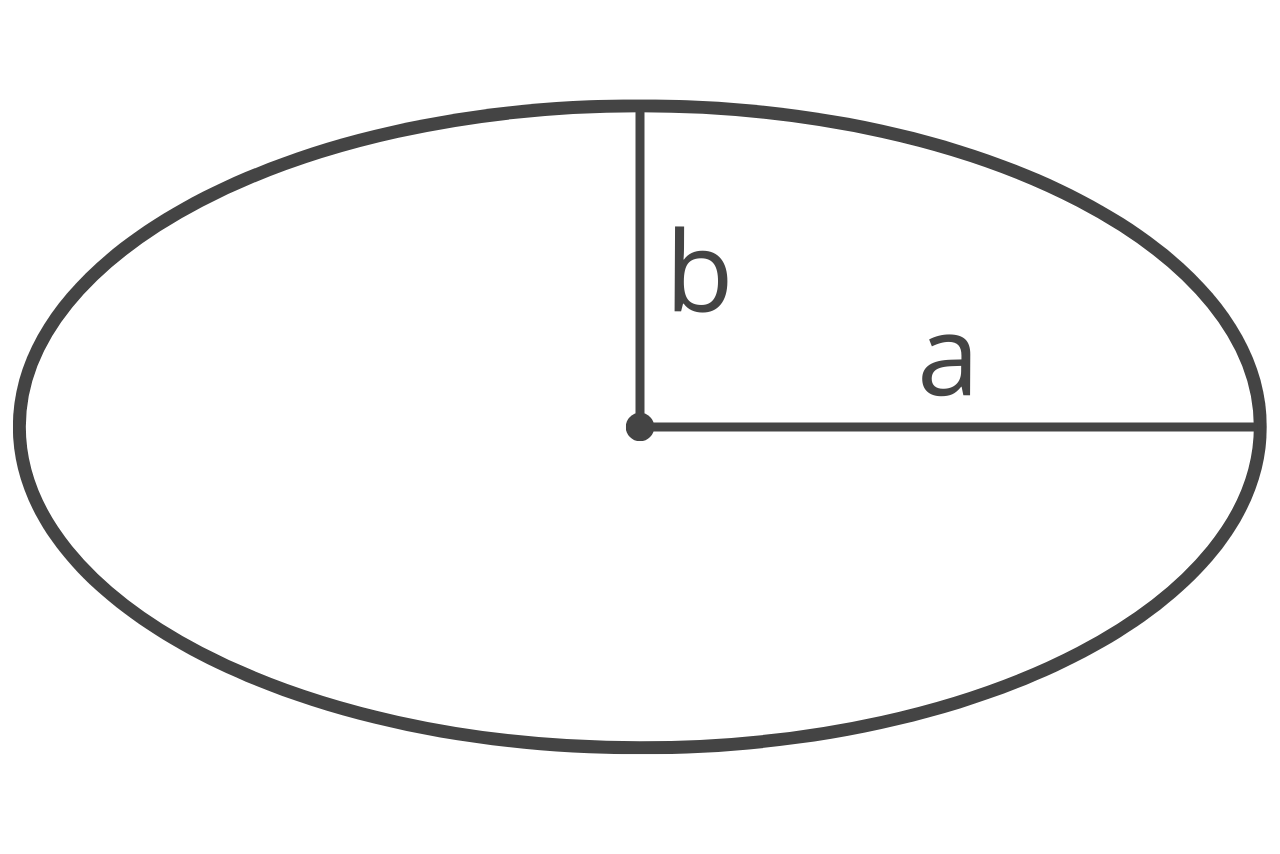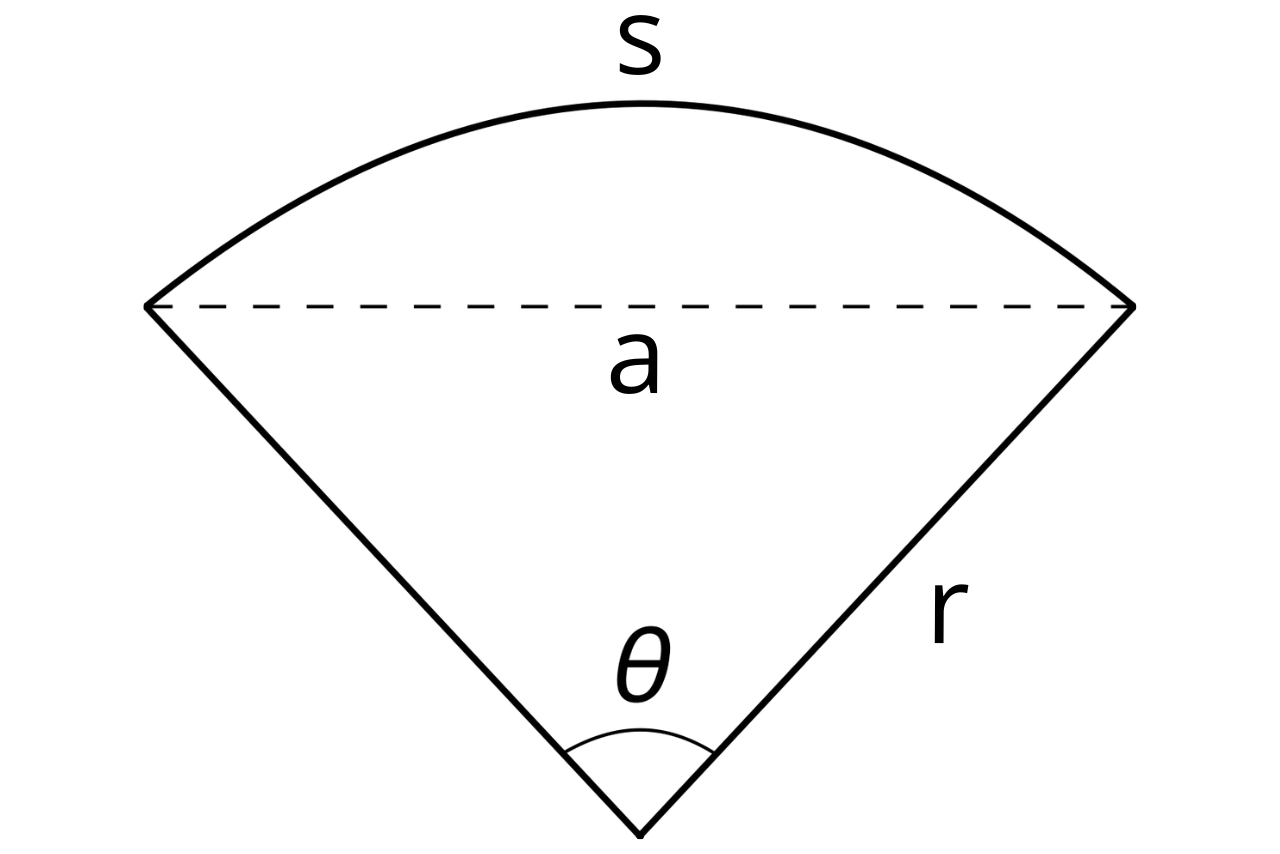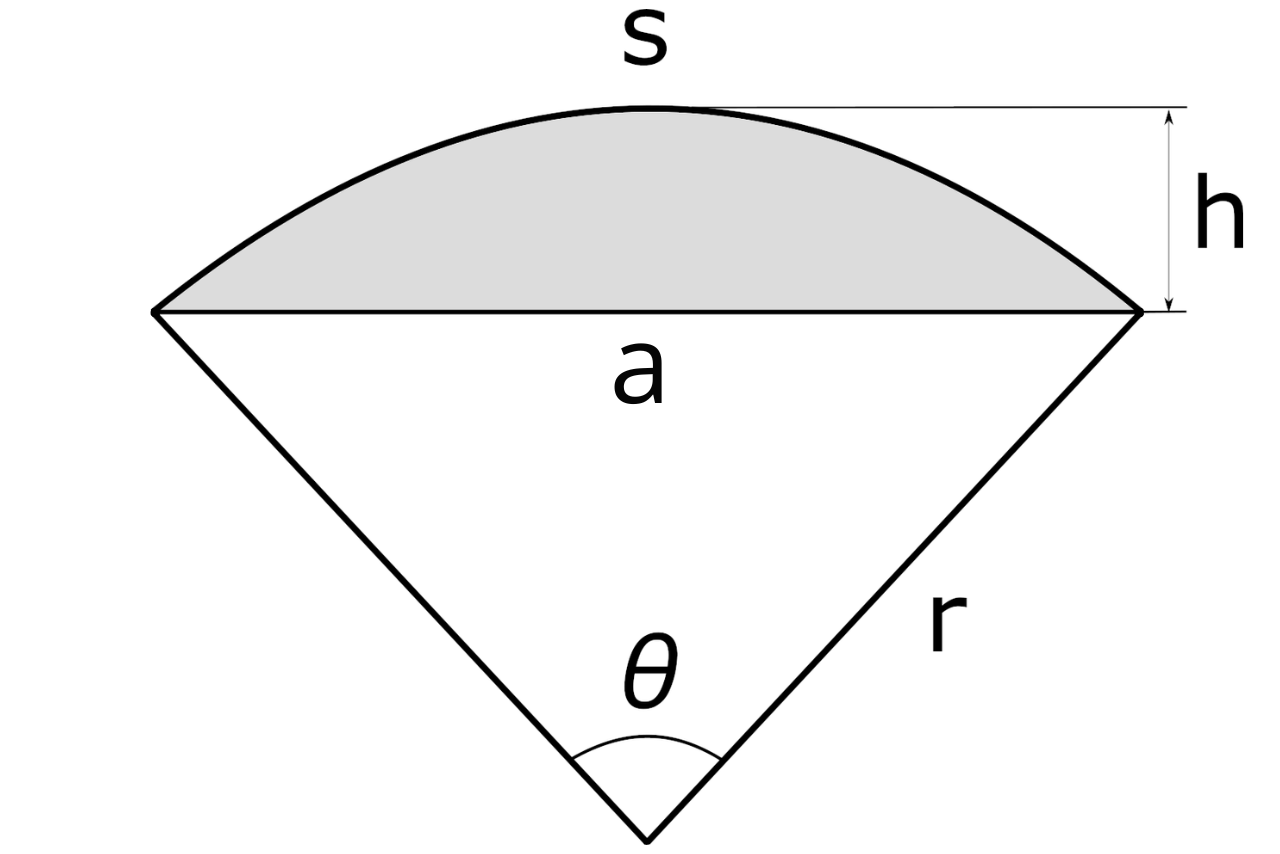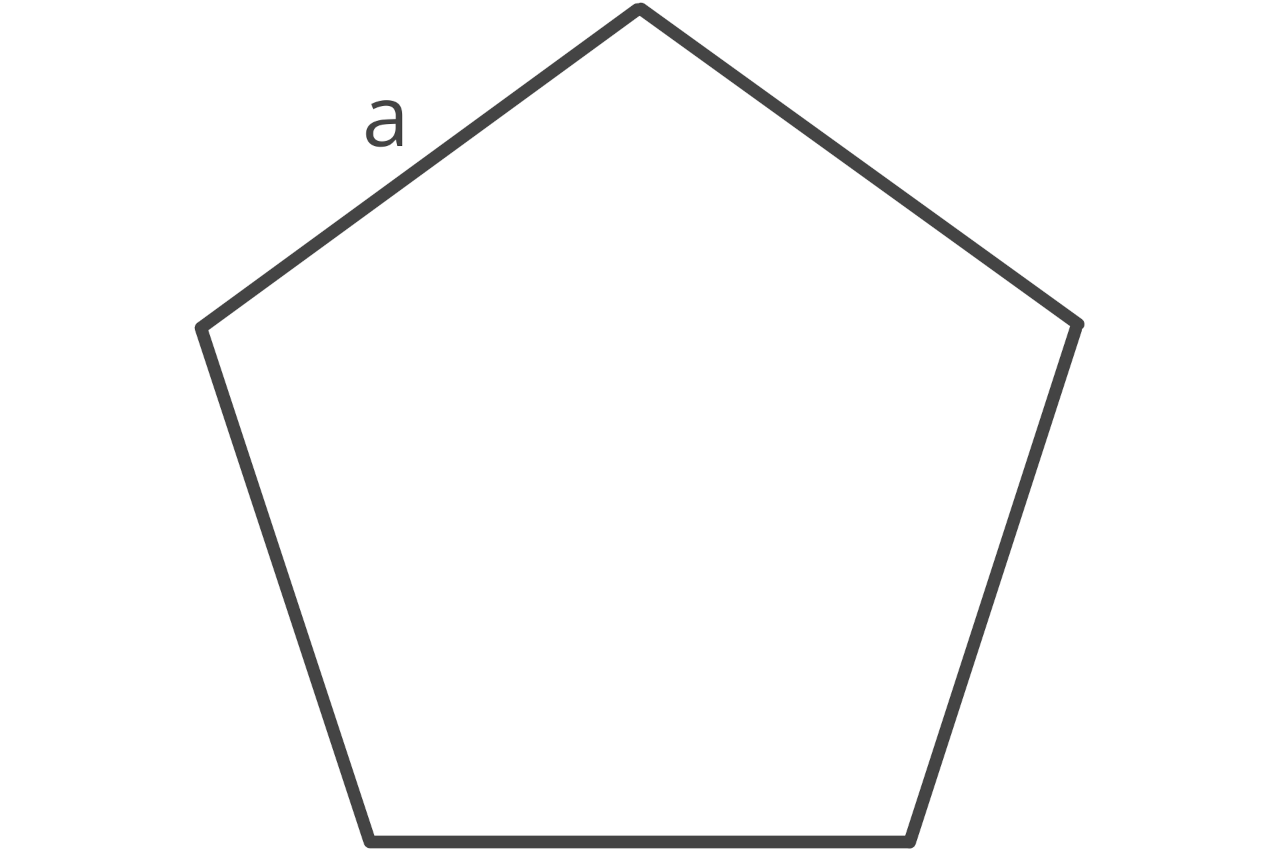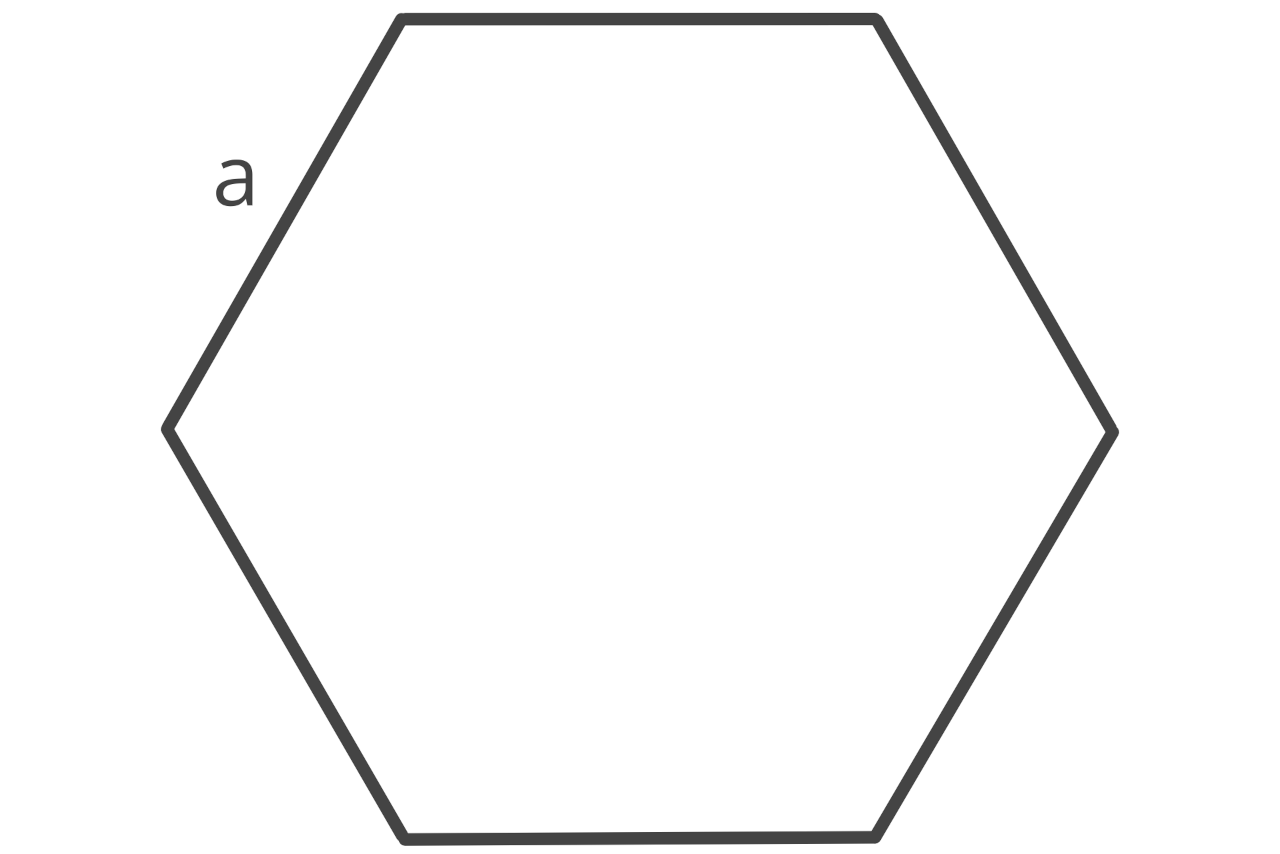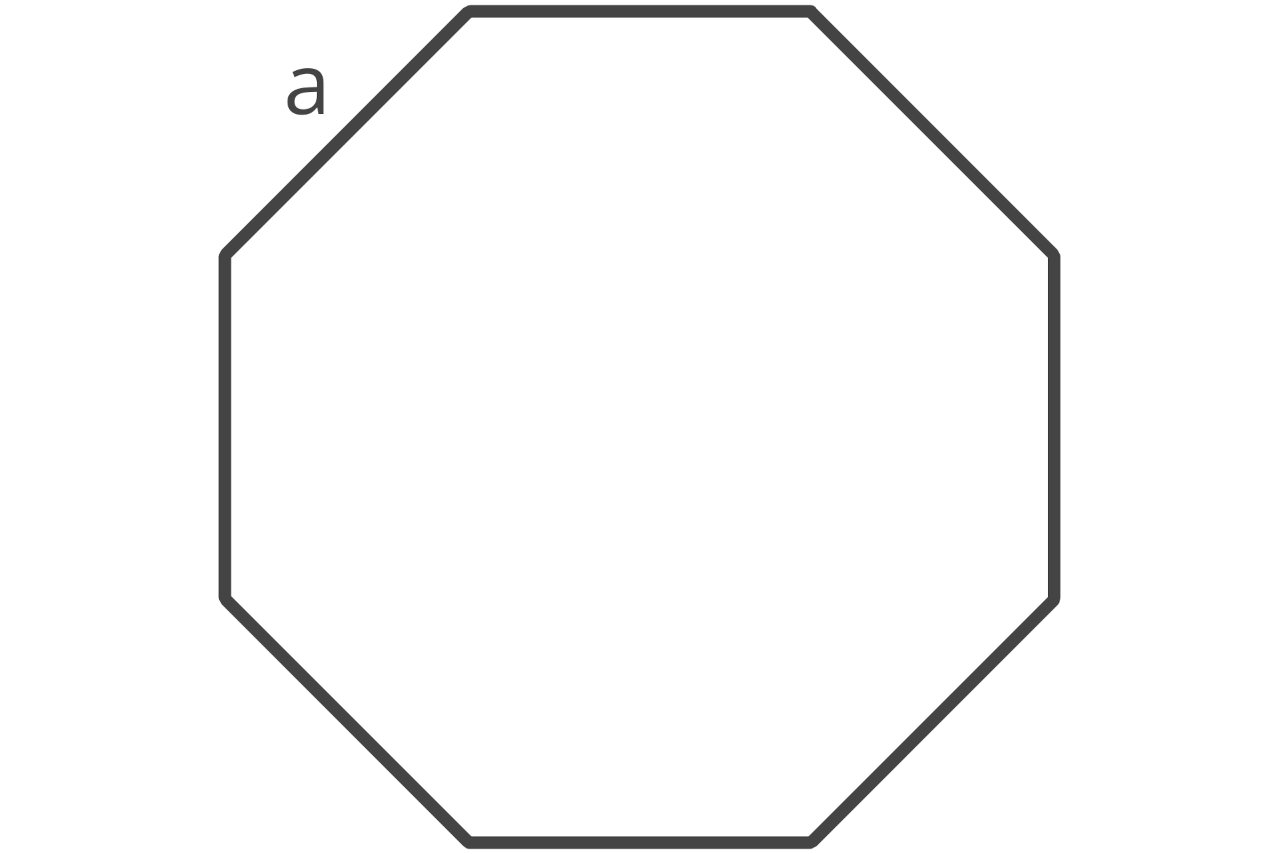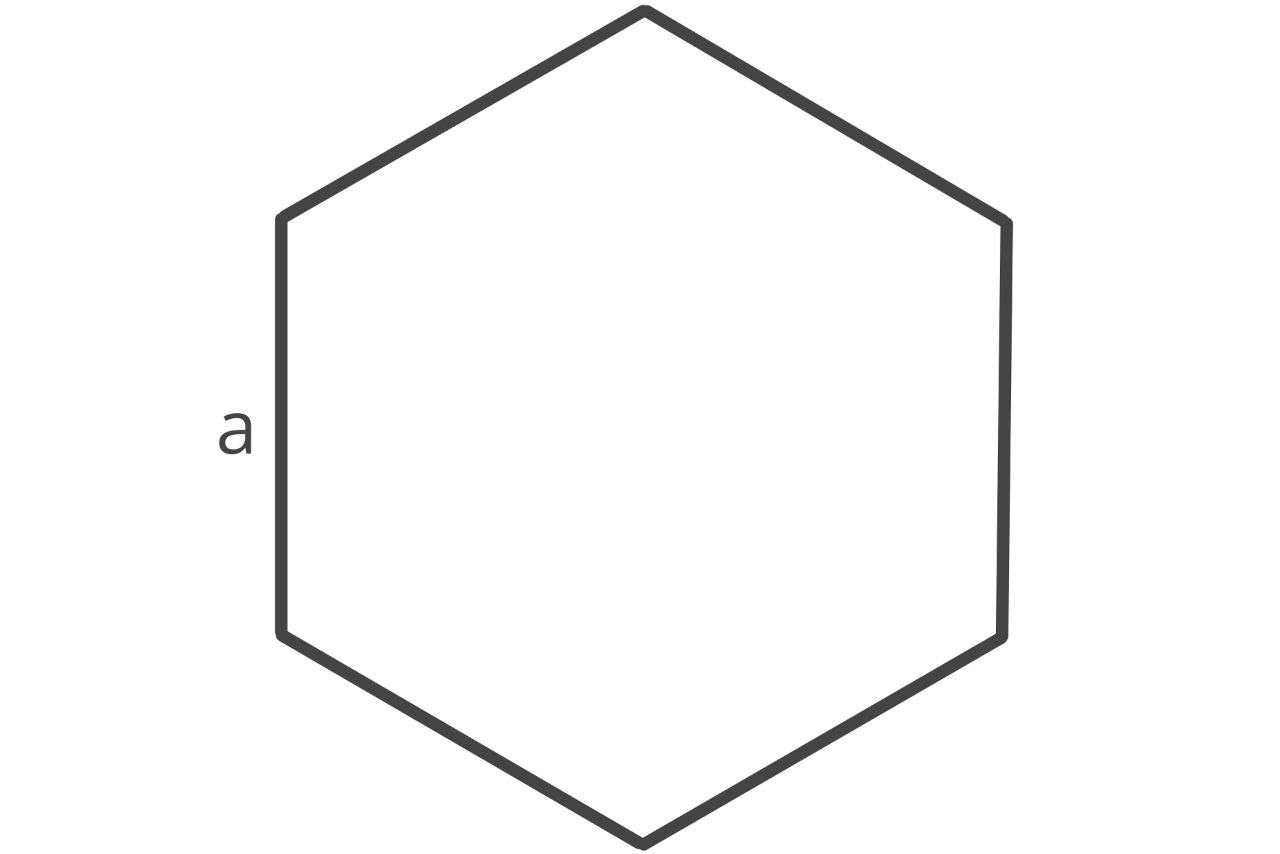## Perimeter Result:

Learn how we calculated this below

## How to Find the Perimeter of a Shape

Perimeter is the length of the outer edge or boundary of a two-dimensional shape and is expressed in units of length, such as inches or feet. The word perimeter is actually derived from the Greek words peri (‘around’) and metron (‘measure’), meaning a measurement around something.

Perimeter is the total boundary length of a shape, meaning it is equal to the sum of the edge lengths for polygons with multiple edges.

You can find the perimeter of any polygon by measuring each edge of the shape and adding the edge lengths together to get the total length. For instance, the perimeter of a rectangle is equal to the length plus the length plus the width plus the width.

For shapes with curved edges, such as a circle or ellipse, the perimeter is referred to as the circumference, and you’ll need a formula to find it.

When measuring, use the same unit of measure for each edge. You can use our length converter to convert all measurements to the same length if needed.

### Perimeter Formulas

Each shape has a unique formula for its perimeter. The formulas for finding the perimeter for the most common shapes are below.

#### Square Perimeter Formula

P = 4a

a = edge length#### Rectangle Perimeter Formula

P = 2l + 2w

l = length
w = width#### Trapezoid Perimeter Formula

P = a + b + c + d

a = base a
b = base b
c = side c
d = side d#### Parallelogram Perimeter Formula

P = 2a + 2b

a = side
b = base#### Rhombus Perimeter Formula

P = 4a

a = edge length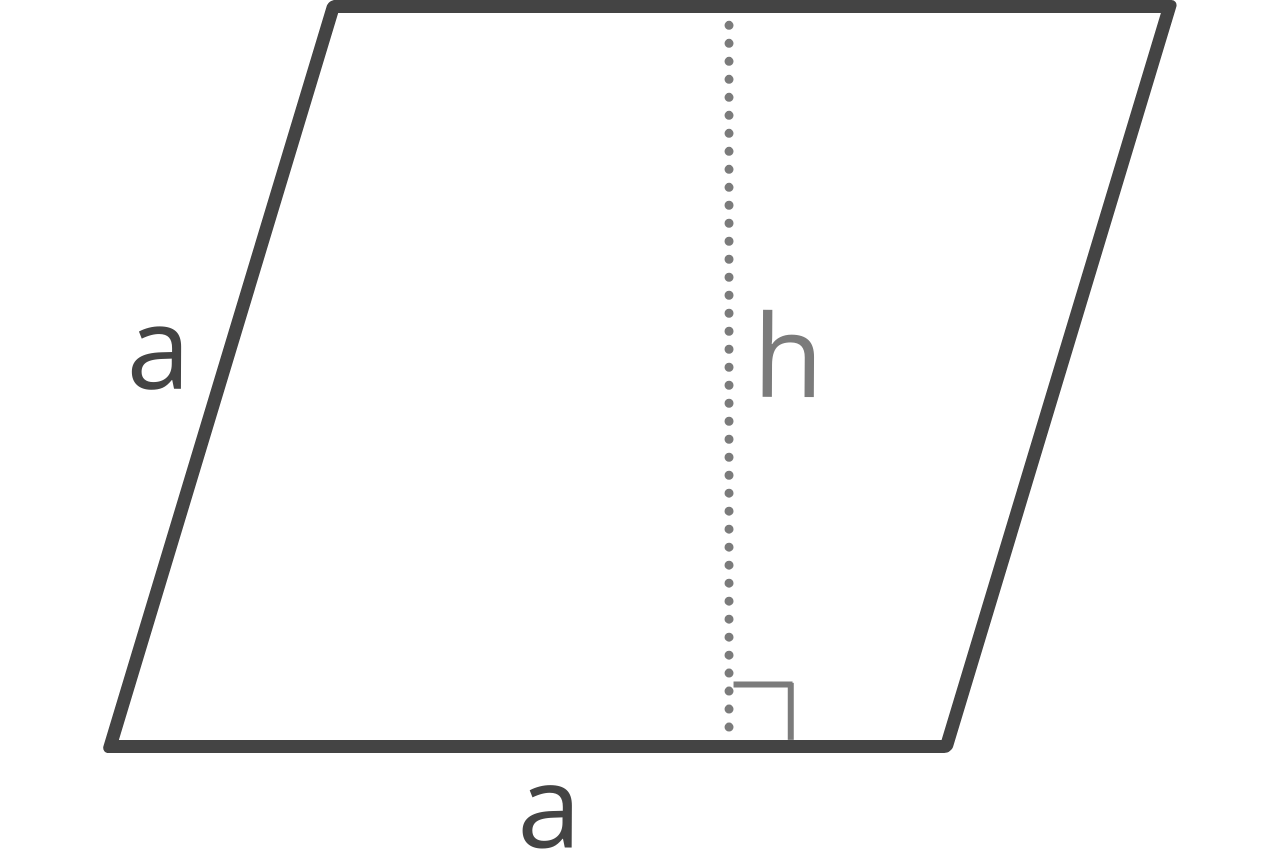#### Triangle Perimeter Formula

P = a + b + c

a = edge a
b = edge b
c = edge c

You can also use our triangle calculator to calculate perimeter using interior angles if all of the sides are not known.#### Circle Perimeter Formula

P = 2πr

Try our circumference calculator to solve using the radius, diameter, or area.#### Ellipse Perimeter Formula

P ≈ π[3(a + b) – (((3a + b))(a + 3b))]

a = axis a
b = axis b#### Sector Perimeter Formula

P = 2r + ((2θπr) ÷ 360)

θ = angle in degrees#### Sector Perimeter Formula

P = πrθ / 180 + 2r × sin(θ / 2)

θ = angle in degrees#### Pentagon Perimeter Formula

P = 5a

a = edge length#### Hexagon Perimeter Formula

P = 6a

a = edge length#### Octagon Perimeter Formula

P = 8a

a = edge length#### Regular Polygon Perimeter Formula

P = an

a = edge length
n = number of sidesTo find the area of these shapes, try our area calculator.

### Is perimeter the same as circumference?

While both perimeter and circumference reference the length of the outer edge or boundary of a shape, perimeter is the measure of the outer edge of shapes with straight sides and circumference is the measure of the outer edge of a circle.

### Is perimeter the same as area?

No, perimeter and area are not the same. Perimeter is the measure of the total distance around a shape and area is the measure of the space inside the shape.

### How do you know if its area or perimeter?

Area is measured in square units while perimeter is not. When measuring area, you multiply a dimension by a dimension, giving you a squared units answer. While with perimeter, you are finding the total length of the outer edge.

### Why do you calculate perimeter?

We calculate perimeter for situations such as knowing how much fencing one would need to fence in a yard, knowing how many strings of Christmas lights you need for the windows of your house, or framing a large painting.